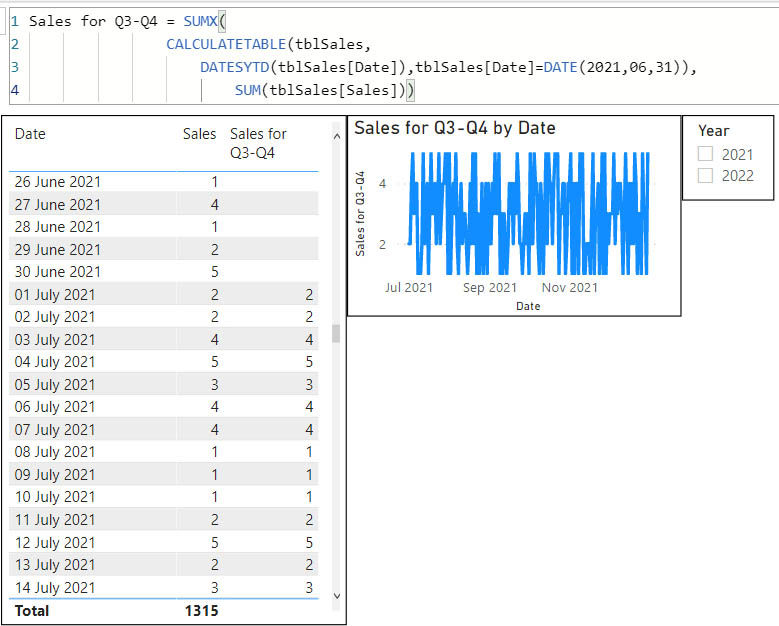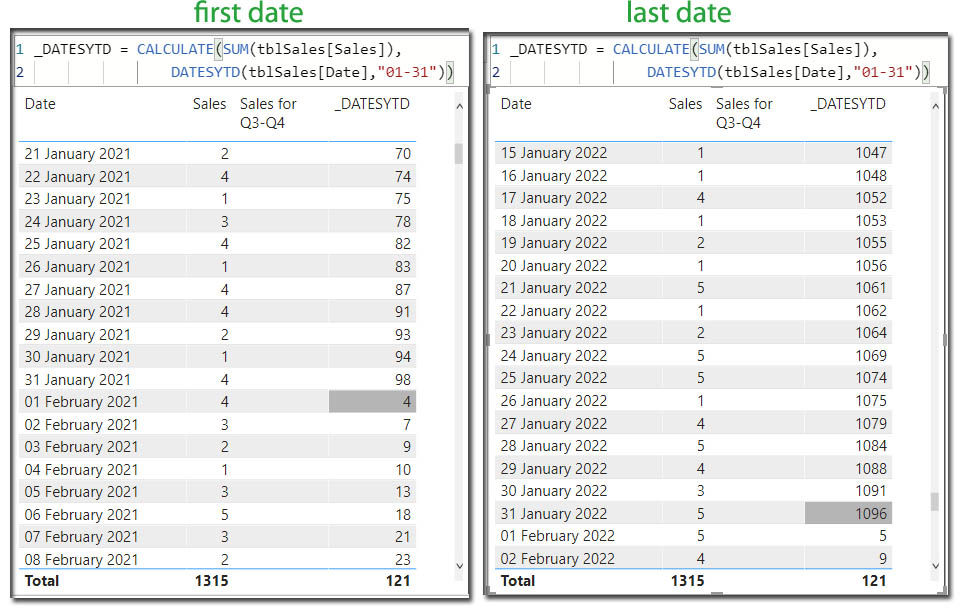top of page
Search
• Marek Vavrovic

# Time intelligence functions, part 02

Updated: Sep 26, 2021

DATESMTD,DATESYTD (cumulative total functions, fiscal year)

syntax

Moves the given set of dates by a specified time interval.

Example 1

calculating Last month sales and variation.

LAST MONTH SALES

Using month granularity. DATEADD(DimDates[Date],-1,MONTH)) shitted all the daily sales one month lower. If you would be using different granularity to display the data, you get a different result backExample 1

DATEADD(DimDates[Date],-1,QUARTER)) is shifting the sales 1 quarter lower.## DATESBETWEEN

Returns the dates between two given dates.

syntax

DATESBETWEEN(<dates>, <start_date>, <end_date>)

### Parameters

Term Definition

dates A date column.

start_date A date expression.

end_date A date expression.

Example

I want to display the Sales for the 1. quarter of 2019.

Quarter 1 2019 Sales = CALCULATE(SUM(FactSales[Sales]), DATESBETWEEN(DimDates[Date],DATE(2019,1,1),DATE(2019,4,30)))Using variables## DATESINPERIOD

Returns the dates from the given period

syntax

DATESINPERIOD(<dates>, <start_date>, <number_of_intervals>, <interval>)

Example

The following expression evaluates the measure Sales Amount in the last 12 months starting from the last day of the period in the filter context.

two measures involved

1> Sales(YTD) = CALCULATE(SUM(FactSales[Sales]),DATESYTD(DimDates[Date]))

2> Sales Moving Annual Total = CALCULATE ( [_Sales(YTD)], DATESINPERIOD ( DimDates[Date], MAX ( DimDates[Date] ), -1, YEAR))Example

This measure start at 31.jun 2020, which is Quarte 2 2020 and goes back to Quarter 2 2019.Example

Calculating 1 year moving average

1 year moving average = CALCULATE( AVERAGE(FactSales[Sales]), DATESINPERIOD(FactSales[Date],LASTDATE(FactSales[Date]),-1,YEAR))## DATESMTD

Returns a set of dates in the month up to the last date visible in the filter context

Cumulative total in each month.

syntax

DATESMTD(<dates>)

Example

DATEMTD creates a group by month and calculates the cumulative total. As I have just 2 records per month, using the dates from the fact table (picture 1) On picture 2 you can see how the data looks like if your fact table is really tiny. But this is not a real life scenario.Example

Calculating the cumulative total in the month group and starting from the quarter 2.Example

In this example I am retrieving the value of the DATESMTD function, the cumulative sum, on some specific date (15.jan.2019)

DATESMTD IS EQUAL TO DATE = CALCULATE( CALCULATE(SUM(FactSales[Sales]), DATESMTD(FactSales[Date])), FactSales[Date]=DATE(2019,1,15))## DATESYTD

Returns a set of dates in the year up to the last date visible in the filter context.

Example

calculate Cumulative running total for the sale column.Example

I want to display the Sales starting from 1st of July 2021

Sales for Q3-Q4 =

SUMX (

CALCULATETABLE (

tblSales,

DATESYTD ( tblSales[Date] ),

tblSales[Date] = DATE (2021, 06, 31)

),

SUM ( tblSales[Sales] ))Example

I want to overwrite the start of the year. I want my first date of the year to be 1. February. This measure comes handy if you need to calculate the fiscal year.

_DATESYTD =

CALCULATE (SUM ( tblSales[Sales] ), DATESYTD ( tblSales[Date], "01-31"))